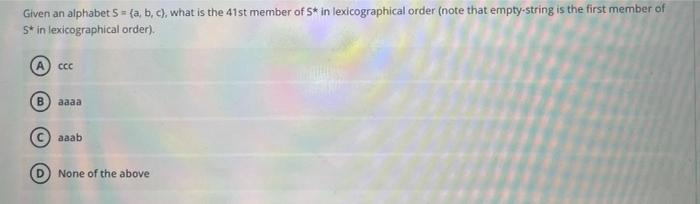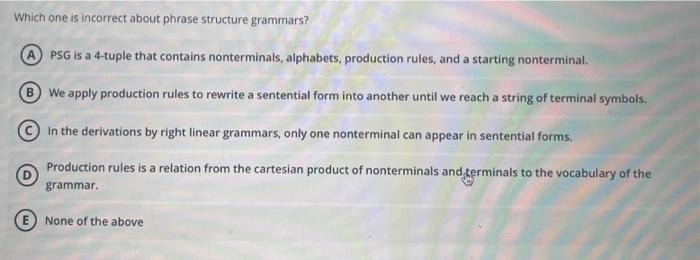Home / Expert Answers / Computer Science / given-an-alphabet-s-a-b-c-what-is-the-41-s-t-member-of-s-in-lexicograp-pa390

# (Solved): Given an alphabet \( S=\{a, b, c\} \), what is the \( 41 s t \) member of \( S^{*} \) in lexicograp ...Given an alphabet \( S=\{a, b, c\} \), what is the \( 41 s t \) member of \( S^{*} \) in lexicographical order (note that empty-string is the first member of 5* in lexicographical order). cec aaaa aaab None of the above Which one is incorrect about phrase structure grammars? PSG is a 4-tuple that contains nonterminals, alphabets, production rules, and a starting nonterminal. We apply production rules to rewrite a sentential form into another until we reach a string of terminal symbols. In the derivations by right linear grammars, only one nonterminal can appear in sentential forms. (D) Production rules is a relation from the cartesian product of nonterminals and therminals to the vocabulary of the grammar. E None of the above

We have an Answer from Expert